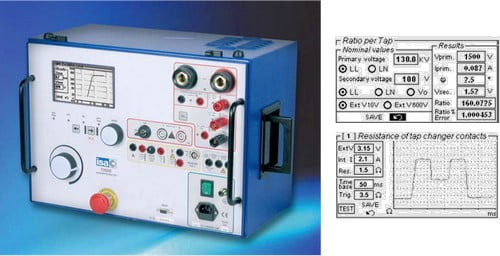# Ratio Test and On-line Tap Changer Test

A ratio test checks the ratio between the secondary and primary voltages for each phase of the transformer. The results are then compared to the nominal plate values. Deviation between the nominal values and the measured values can indicate a fault such as:

• A short within a coil
• A short between HV coil and LV coil – this will be shown as a very significant deviation
• A short through the insulation or the tap changer.

A ratio tests needs to be done when the transformer is disconnected from its cable system. The ratio tester injects a known voltage to the primary coil and measures the voltage in the secondary coil, extracting the ratio (and considering the connection group, the result may need to be divided by root of 3).

The disadvantage of a ratio test is that the fault must be mirrored to a ratio test. Faults such as mechanical deformation in the coils or loose connections will not be shown. Sweep Frequency Response Analysis test can detect these faults.

Ratio tests are also used to measure the correct operation of the tap changer. Moving the tap changer from one position to another and reapplying the ratio test should yield the expected ratio from each tap position.